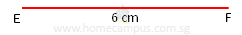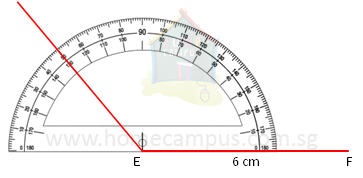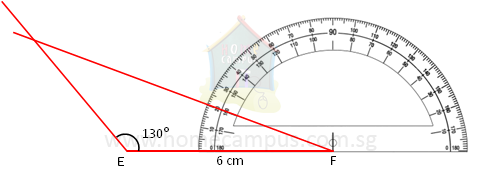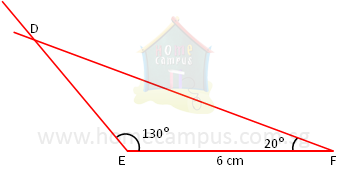## How to Draw Triangles using Geometrical Instruments? - Part 3

Practice Unlimited Questions

#### 1. Draw a triangle DEF with ∠DEF = 130°, ∠DFE = 20° and EF = 6 cm.

Step 1: Draw a straight line EF 6 cm long.Step 2: Using a protractor draw an angle of 130° from Point E.Step 3: Using a protractor draw an angle of 20° from Point F.Step 4: Mark the point where the two lines meet as Point D.Δ DEF is the required triangle.